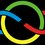# Problem 5! IMO 2015

Let $\mathbb{R}$ be the set of real numbers. Determine all functions $f:\mathbb{R}\to\mathbb{R}$ that satisfy the equation

$f(x+f(x+y))+f(xy)=x+f(x+y)+yf(x)$

for all real numbers $x$ and $y$.

##### This is part of the set IMO 2015Note by Sualeh Asif
5 years, 2 months ago

This discussion board is a place to discuss our Daily Challenges and the math and science related to those challenges. Explanations are more than just a solution — they should explain the steps and thinking strategies that you used to obtain the solution. Comments should further the discussion of math and science.

When posting on Brilliant:

• Use the emojis to react to an explanation, whether you're congratulating a job well done , or just really confused .
• Ask specific questions about the challenge or the steps in somebody's explanation. Well-posed questions can add a lot to the discussion, but posting "I don't understand!" doesn't help anyone.
• Try to contribute something new to the discussion, whether it is an extension, generalization or other idea related to the challenge.

MarkdownAppears as
*italics* or _italics_ italics
**bold** or __bold__ bold
- bulleted- list
• bulleted
• list
1. numbered2. list
1. numbered
2. list
Note: you must add a full line of space before and after lists for them to show up correctly
paragraph 1paragraph 2

paragraph 1

paragraph 2

[example link](https://brilliant.org)example link
> This is a quote
This is a quote
    # I indented these lines
# 4 spaces, and now they show
# up as a code block.

print "hello world"
# I indented these lines
# 4 spaces, and now they show
# up as a code block.

print "hello world"
MathAppears as
Remember to wrap math in $$ ... $$ or $ ... $ to ensure proper formatting.
2 \times 3 $2 \times 3$
2^{34} $2^{34}$
a_{i-1} $a_{i-1}$
\frac{2}{3} $\frac{2}{3}$
\sqrt{2} $\sqrt{2}$
\sum_{i=1}^3 $\sum_{i=1}^3$
\sin \theta $\sin \theta$
\boxed{123} $\boxed{123}$

Sort by:

It is not very hard to see that f(x) = x and f(x) = 2 - x are solutions. The difficult part is to show that they are the only solutions. In my eyes, Sualeh Asif demonstrates that f(0) = 2 implies that f(x) = x - 2. My compliments! But I am not convinced by his reasoning that f(0) = 0 implies that f(x) = x for all real x. In particular, I do not see the consequence of P(x,1) on line 2 of case 2. Neither the consequence of P(1,f(x+1)+x) on line 4 of case 2.

- 5 years ago

Let $P(x,y)$ be the above FE.

$P(0,0)\implies f(f(0))+f(0)=f(0)\implies f(f(0))=0$ $P(0, f(0))\implies 2f(0)=f(f(0))+f(0)^2\implies f(0)^2-2f(0)=0\implies f(0)=0, 2$ Case 1: $f(0)=0$. Then $P(0, y)\implies f(f(y)) + f(0)=f(y)+yf(0)\implies f(f(y))=f(y)$ Since the range of $f:\mathbb{R}\to\mathbb{R}$ is $\mathbb{R}$, then $\boxed{f(x)=x}$.

Case 2: $f(0)=2$. Then let $g(x)=f(x)-2$ which changes the FE to $P(x):= g(g(x+y)+x+2)+f(xy)+2=x+g(x+y)+yg(x)+2y$ [I'll finish later]

- 5 years, 2 months ago

It does look like $f(x) = 2-x$ satisfies the functional equation. My guess would be $f(x) = x$ or $f(x) = 2-x$, but I do agree with the comment that case 1 still has some work to be done to get to $f(x) = x$.

- 5 years, 2 months ago

This problem is quite a bit harder than I perceived it to be. I don't think I can find a solution.

- 5 years, 2 months ago

Daniel Liu The problem is one of those bash types on the IMO where if you keep bashing long enough you do end up on a solution! You were on the right track! Here is a solution from AOPS (by wanwan4343) which bashed in the same way! I essentially also took the same path too and ended up on the functions! (with a somewhat incomplete proof):

Let $P(x,y)$ be the assertion $f(x+f(x+y))+f(xy)=x+f(x+y)+yf(x)$

$P(0,0)\Rightarrow f(f(0))=0 \\P(0,f(0))\Rightarrow 2f(0)=f(0)^2\Rightarrow f(0)=0 \text{or} 2$

case 1.

$f(0)=2\Rightarrow f(2)=0 \\ P(x,1)\Rightarrow f(x+f(x+1))=x+f(x+1) \\ P(0,f(x+1)+x)\Rightarrow f(x+1)+x+2=f(x+1)+x+2(f(x+1)+x) \\ \Rightarrow f(x)=2-x\forall \in\mathbb{R}$

Case 2.$f(0)=0$

$P(x,0)\Rightarrow f(x+f(x))=x+f(x) \\P(x,1)\Rightarrow f(x+f(x+1))=x+1+f(x) \\ P(1,f(x+1)+x)\Rightarrow f(1+f(1+x+f(x+1)))+f(x+f(x+1))=1+f(x+1+f(x+1))+f(x+1)+x \\ \Rightarrow f(f(x)+x+1)=f(x)+x+1 \\ P(x,-1)\Rightarrow f(x+f(x-1))+f(-x)=x+f(x-1)-f(x) \\ \Rightarrow -f(x)=f(-x)\\ P(x,-x)\Rightarrow f(x)+f(-x^2)=x-xf(x)\\ P(-x,x)\Rightarrow f(-x)+f(-x^2)=-x+x(-x) \\ \Rightarrow f(x)-f(-x)=2x-x(f(x)+f(-x)) \\ \Rightarrow f(x)=x \forall x\in\mathbb{R}$

hence, $f(x)=2-x$ and $f(x)=x$ are solutions.

- 5 years, 2 months ago

Oh dang, I never thought of putting something as unusual as $f(x+1)+x$ in the FE.

- 5 years, 2 months ago

That is what most people never thought! @Daniel Liu and that is why this is problem 5 of the IMO!

- 5 years, 2 months ago

Okay, so Both problems #2 and #5 were bashy. :(

- 5 years, 2 months ago

Yeah that is what I also saw! A bashing Algebra and NT problem!

However problems #1, #4 and #6 have been generally appreciated

- 5 years, 2 months ago

Yes you are right @Patrick Corn !

$f(x)= x$ or $2-x\quad \forall x$

- 5 years, 2 months ago

In case 1, you must prove that $f$ is surjective to make that conclusion because $\mathbb R$ is the codomain of $f$, which is not necessarily the range.

- 5 years, 2 months ago